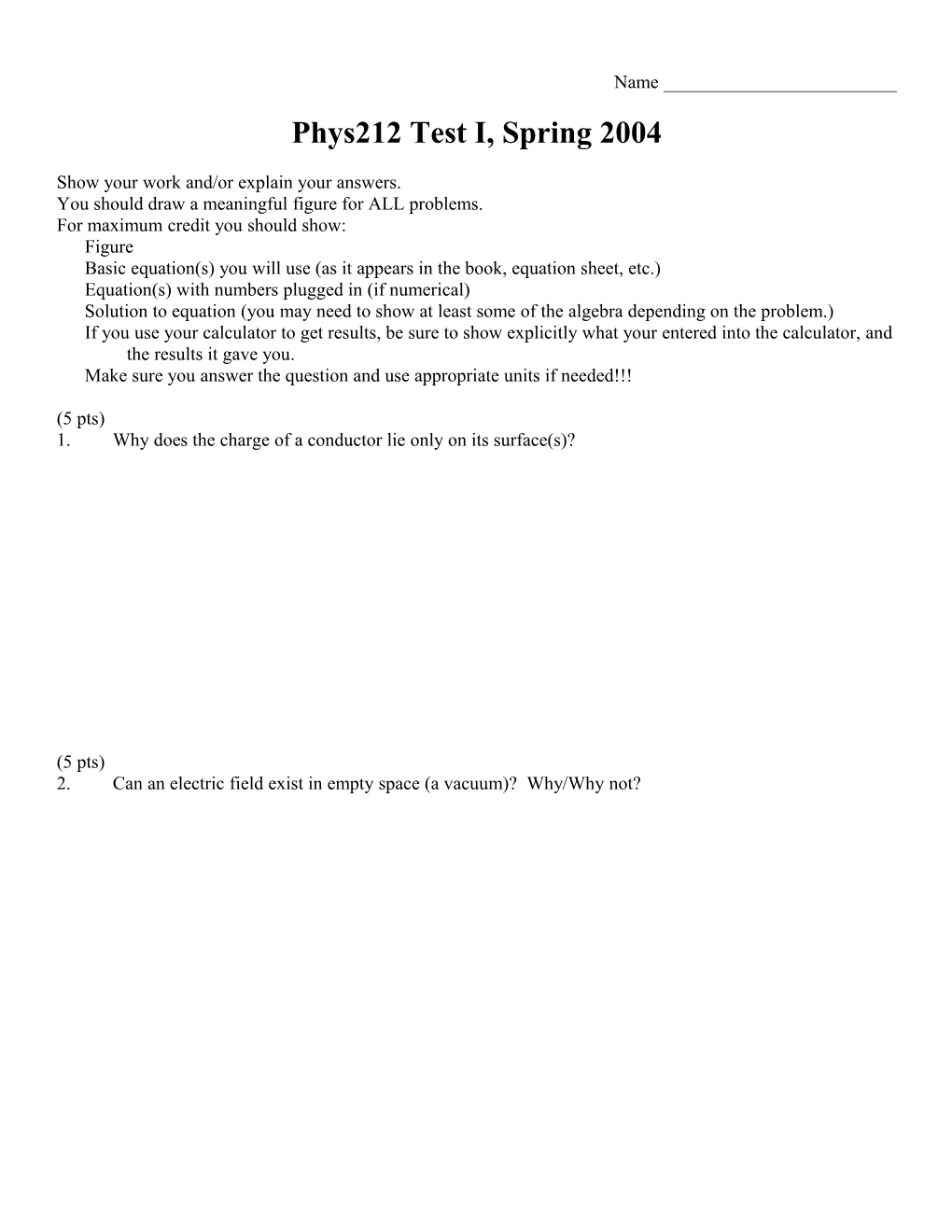# Phys 150 Sample Test I, Fall 1997Name ______

Phys212 Test I, Spring 2004

You should draw a meaningful figure for ALL problems.

For maximum credit you should show:

Figure

Basic equation(s) you will use (as it appears in the book, equation sheet, etc.)

Equation(s) with numbers plugged in (if numerical)

Solution to equation (you may need to show at least some of the algebra depending on the problem.)

If you use your calculator to get results, be sure to show explicitly what your entered into the calculator, and the results it gave you.

Make sure you answer the question and use appropriate units if needed!!!

(5 pts)

1.Why does the charge of a conductor lie only on its surface(s)?

(5 pts)

2.Can an electric field exist in empty space (a vacuum)? Why/Why not?

(4 pts each) For #3-#7, circle the best answer.

3.Two charges are fixed in the positions shown. Where could a third charge be placed so that it has no net force acting on it?

ABCDE

4.Four Coulombs of charge is spread uniformly on the surface of a plane measuring 2m x 1m. What is the most appropriate density to describe this charge distribution?

A:  = 2 C/m2D:  = 4 CG:  = 8 Cm2

B:  = 2 C/m2E:  = 4 CH:  = 8 Cm2

C:  = 2 C/m2F:  = 4 CI:  = 8 Cm2

5.Suppose you are applying Gauss’ Law to analyze an infinite line charge. What shape of a Gaussian surface should be used?

## A: CircleD: Line

B: CubeE: Plane

C: CylinderF: Sphere

6.In the figure below, there is an unknown charge at the center of a thick spherical shell. The total charge on the inside wall and the outside wall of the shell are given. What is the charge at the center?A:7 nCH:-7 nC

B:6 nCI:-6 nC

C:5 nCJ:-5 nC

D:4 nCK:-4 nC

## F:2 nCM:-2 nC

G:1 nCN:-1 nC

7.Which is the most correct figure showing the electric field lines from a line segment?

ABCDEF

(11pts)

8.A 9 mC charge is fixed to a table top so that it cannot move. A small 20 g object with a charge of –2 nC is then placed 28 cm above the fixed charge. What additional electric field is needed to make the 20 g object be in equilibrium? (don’t forget about gravity)

(11pts)

9.A spherical insulator of radius R has a uniform charge density  throughout its volume. There is also a negative point charge of –Q located at the center of the sphere.

Use Gauss’ Law to find the electric field at a point P which is outside the sphere.(11pts)

10.A charge of Q is uniformly spread around a ring of radius R. Derive the electric field at a point P which lies on the axis of the ring a distance d from the center of the ring? You must start with

,

and be sure to draw a complete figure to support your work.